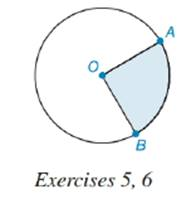Chapter 8.5, Problem 5EElementary Geometry For College St...

7th Edition
Alexander + 2 others
ISBN: 9781337614085

Solutions

Chapter
SectionElementary Geometry For College St...

7th Edition
Alexander + 2 others
ISBN: 9781337614085
Textbook Problem

In the circle, the radius length is 10 in. and the length of A B ^ is 14 in. What is the perimeter of the shaded sector?To determine

To find:

The perimeter of shaded sector.

Explanation

Definition:

Perimeter is the distance around a two dimensional shape.

The distance around the sector is the sum lengths of two radii and the arc intercepted by them.

Formula:

If r is the radius of circle,

Psector=r+r+lintercepted arc=2r+lintercepted arc

Thus, Psector=2r+lintercepted arc

Calculation:

It is given that radius of circle measures 10 in. Thus, r=10.

The arc intercepted by radii OA and OB is AB^

Still sussing out bartleby?

Check out a sample textbook solution.

See a sample solution

The Solution to Your Study Problems

Bartleby provides explanations to thousands of textbook problems written by our experts, many with advanced degrees!

Get Started

Expand each expression in Exercises 122. (4+2x)(42x)

Finite Mathematics and Applied Calculus (MindTap Course List)

Evaluate the integral. 63. xexdx

Single Variable Calculus: Early Transcendentals

The tangential component of acceleration for at t = 0 is: 0

Study Guide for Stewart's Multivariable Calculus, 8th

f(x) = x3 2x2 + x 1 has a local maximum at: a) 0 b) 1 c) 13 d) f has no local maxima

Study Guide for Stewart's Single Variable Calculus: Early Transcendentals, 8th